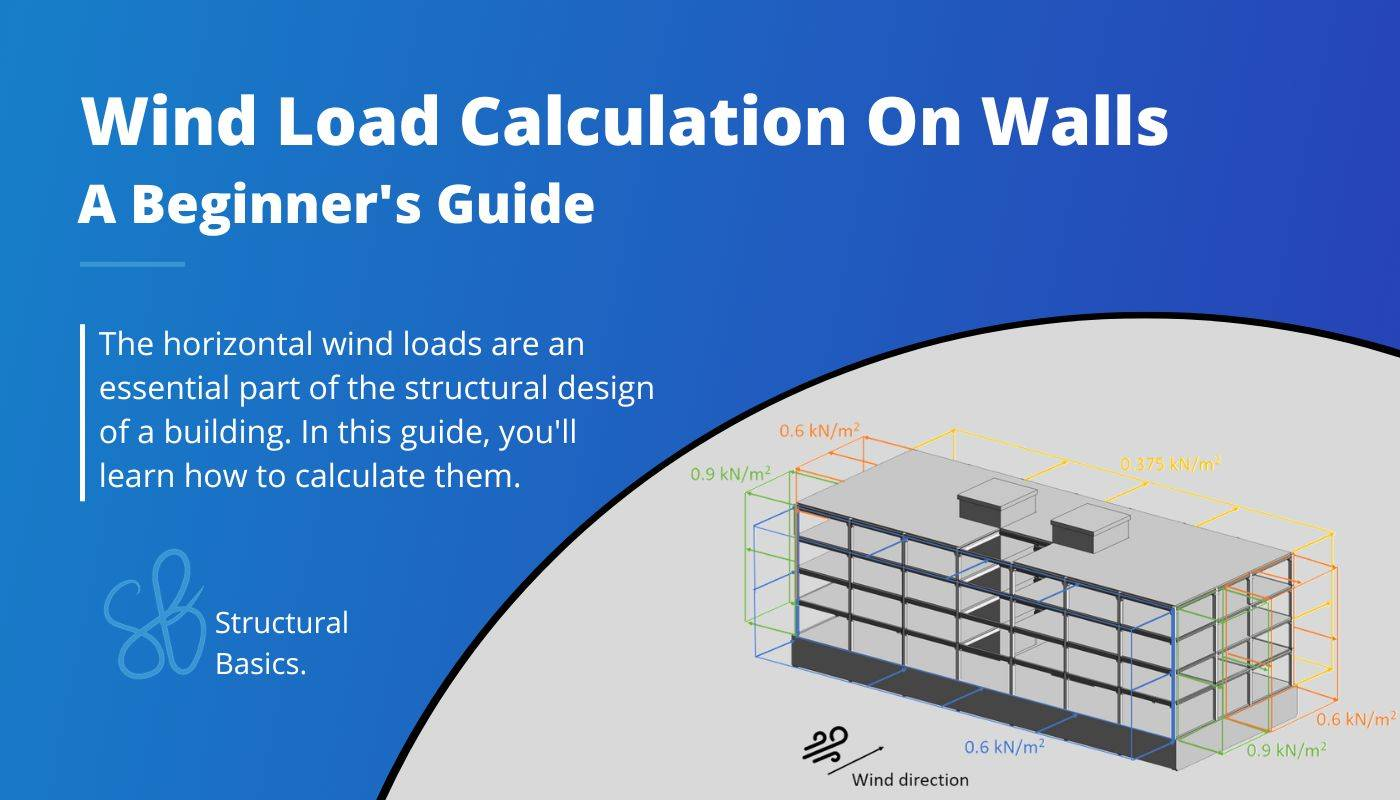# Wind Load Calculation On Walls [A Beginner’s Guide]Last updated: July 19th, 2023

We like to explain it with examples. This time, we will calculate the wind forces on facade elements for a precast concrete office building.

The following picture shows only the structural elements.

The calculation of the wind load is split up in multiple articles due to the fact that the wind load depends on much more parameters that need to be derived than for example the snow load.

In order to calculate the wind load or wind pressure on external surfaces, we are going to do the following steps:

1. Calculate the wind velocity pressure $q_{p}$
2. Define the outer geometry of the building
3. Calculate the width of the Wind areas
4. Find the external pressure coefficients

## Define geometry and parameters from wind velocity pressure

This is a quick summary of the values we calculated to get the wind velocity pressure.

## Wind pressure on surfaces

### Wind pressure on external surfaces $w_{e}$

The formula to calculate the wind pressure on external surfaces is

$w_{e} = q_{p} * c_{pe}$

Where

The coefficient $c_{pe}$ has 2 different values depending on the wind loaded area.

There is a value for a surface area of 1 $m^2$ and 10 $m^2$. These to values can also be written as

You are now probably wondering: “When do we ever design a load bearing element where only 1 $m^2$ is loaded?🤔”

Well, you are right, that is almost never the case for beams and slabs. But for smaller elements like cladding elements of the facade or fixings, $c_{pe.1}$ comes into play (EN 1991-1-4 7.2.1 Note 1).

So as a short summary:

• $c_{pe.10}$ is usually used for the overall load bearing structure
• $c_{pe.1}$ is used for small elements within elements, such as cladding

Enough explanation, let’s have a look at the values of the coefficients.

EN 1991-1-4 Table 7.1 gives recommendations for $c_{pe.10}$ and $c_{pe.1}$. This means that you have to double-check with your National annex because those values might be defined differently there.

Table 7.1 gives values for 5 different areas A, B, C, D and E of our building.

Those areas depend on where the wind comes from and can be seen in EN 1991-1-4 Table 7.1. For our office building, we can define the areas as

### Wind from Front

From those dimensions we can define $e$ which determines the width of Areas A and B according to EN 1991-1-4 Figure 7.5

$e = min(b, 2h)$

$e = min(49.75m, 2*17.1m=34.4m) = 34.4m$

For the case of $e > d$ which is true for us the width of Area A is defined as

$e/5 = 6.9m$

So let’s visualize all of those numbers 😁

We did not include the walls on the roof top in the following picture due to better visualization. We also need to take them into account.

Okay, now let’s go back to the formula for the wind pressure for external surfaces and derive the values. The peak velocity pressure was calculated in the last article as

$q_{p} = 0.746 \frac{kN}{m^2}$

The external pressure coefficients for rectangular buildings can be taken from EN 1991-1-4:2005 Table 7.1.

For a height to width ratio h/d = 17.1m/20.2m = 0.85 which is < 1 and > 0.25 we get the following coefficients.

Based on our coefficients we can now calculate the Wind pressure on external surfaces.

When you calculate the wind loads the first time ever, it might be very confusing in which direction you have to apply the loads.

So let’s apply the wind loads (for 10 $m^2$) on our building.

To better understand it we take a look at a 3D picture.

Now we also have to do the same for the case that wind comes from the side.

### Wind from Side

In the scenario that wind comes from the side we have to define the Area widths again. We have to redefine the geometry parameters.

From those dimensions we can define $e$ according to EN 1991-1-4 Figure 7.5

$e = min(b, 2h)$

$e = min(20.2m, 2*17.1m=34.4m) = 20.2m$

For the case of $e < d$ which is true for us the width of Area A is defined as

$e/5 = 4.04m$

The Area B is calculated as

$e*4/5 = 16.16m$

The Area C is therefore the rest of d and calculated as

$d – e = 49.75m – 20.2m = 29.55m$

So let’s visualize all of those numbers 😁

For a height to width ratio h/d = 17.1m/49.75m = 0.34 which is < 1 and > 0.25 we get the following coefficients we get the same coefficients for A, B, D and E as for Wind from Front but additionally we get now a value for C (EN 1991-1-4:2005 Table 7.1).

Based on our coefficients, we can now calculate the Wind pressure on external surfaces.

Those wind area loads we can now visualize in a floor plan.

## Reduction of Wind force on Areas D and E

According to EN 1991-1-4 7.2.2 (3) the wind loads can be reduced in the direction of the wind (Areas D and E) if they meet the following criteria

$h/d <1$

### Wind from Front

$h/d = 17.1m/20.2m = 0.85 < 1$

The criteria are met, and the forces in Areas D and E can be reduced by the factor 0.85.

### Wind from Side

$h/d = 17.1m/49.75m = 0.34 < 1$

The criteria are met, and the forces in Areas D and E can be reduced by the factor 0.85.